# Assignments

The following assignments are about solving quadratic equations. One method to solve this type of equation is the use of the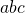-method. This may not always be possible and then other methods such as factorization (Quadratic equations (factorizing)) are possible.

1. Solve:Solution

2. Solve:Solution

3. Solve: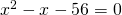Solution

4. Solve: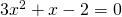Solution

5. Solve: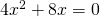Solution

6. Solve: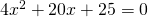Solution

7. Solve: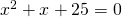Solution

8. For which value(s) of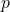does the equation:have two different solutions.

Solution

9. For which value(s) of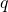does the equation: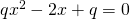have two coinciding solutions.

Solution

10. For which value(s) ofdoes the equation::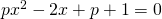have no solutions.

Solution

0